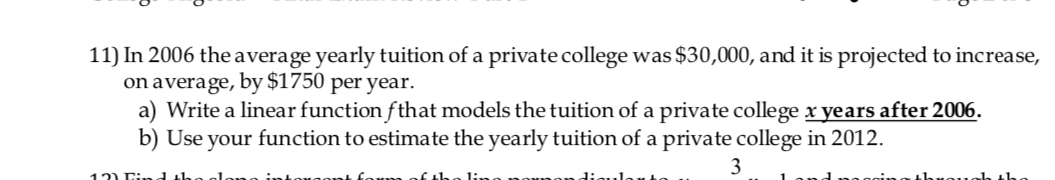11) In 2006 the average yearly tuition of a private college was \$30,000, and it is projected to increase,on average, by \$1750 per year.a) Write a linear function fthat models the tuition of a private college x years after 2006.b) Use your function to estimate the yearly tuition of a private college in 2012.

Questionhelp_outlineImage Transcriptionclose11) In 2006 the average yearly tuition of a private college was \$30,000, and it is projected to increase, on average, by \$1750 per year. a) Write a linear function fthat models the tuition of a private college x years after 2006. b) Use your function to estimate the yearly tuition of a private college in 2012. fullscreen
Step 1

Let x represents the number of years after 2006 and \$ y  represents the yearly tuition fees, When x=0 , y=\$30000 and when x=1, y=\$30000+1750=\$31750 , then the linear function passes through (0,30000) and (1, 31750), From this we can slope of line and the equation of line as shown on board.

Step 2

In 2012, that is when x=6 years, we can find tuitio...

Want to see the full answer?

See Solution

Want to see this answer and more?

Our solutions are written by experts, many with advanced degrees, and available 24/7

See Solution
Tagged in

Algebra